Courses

# Test: Transistor Biasing & Thermal Stabilization

## 10 Questions MCQ Test Topicwise Question Bank for Electronics Engineering | Test: Transistor Biasing & Thermal Stabilization

Description
This mock test of Test: Transistor Biasing & Thermal Stabilization for Electrical Engineering (EE) helps you for every Electrical Engineering (EE) entrance exam. This contains 10 Multiple Choice Questions for Electrical Engineering (EE) Test: Transistor Biasing & Thermal Stabilization (mcq) to study with solutions a complete question bank. The solved questions answers in this Test: Transistor Biasing & Thermal Stabilization quiz give you a good mix of easy questions and tough questions. Electrical Engineering (EE) students definitely take this Test: Transistor Biasing & Thermal Stabilization exercise for a better result in the exam. You can find other Test: Transistor Biasing & Thermal Stabilization extra questions, long questions & short questions for Electrical Engineering (EE) on EduRev as well by searching above.
QUESTION: 1

Solution:
QUESTION: 2

### In a BJT, if the collector junction is reverse biased and the emitter junction is reverse - biased, it is said to operate in

Solution: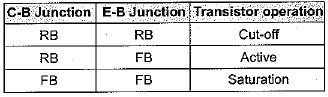QUESTION: 3

### On applying a positive voltage signal to the' base of a normally biased n-p-n CE transistor amplifier

Solution:
QUESTION: 4

Assertion (A): The process of giving proper supply voltages and resistances for obtaining the desired Q-point is called biasing.
Reason (R): In order to produce distortion free output in amplifier circuits, the supply voltages and resistances in the circuit must be suitably chosen.

Solution:
QUESTION: 5

Match List-I (Transistor parameters) with List-ll (Temperature effect) and select the correct answer using the codes given below the lists:
List-I
A. Base-emitter voltage (VBE)
B. Transistor current gain (β)
C. Reverse saturation current (Ico)

List-ll
1. Independent of temperature
2. Decreases by 2.5 mV per oC
3. Increases with temperature
4. Increase by 7% per oC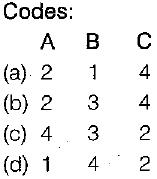Solution:
QUESTION: 6

For the transistor circuit shown below, the Q-point values are given as ICQ = 1 mA and VCEQ = 6V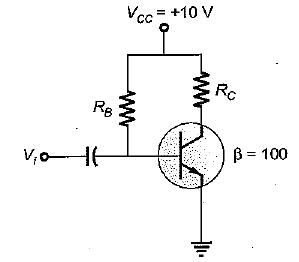If VBE (on) = 0.7 V, the values of the RB and Rc will be respectively

Solution:

The collector resistance is: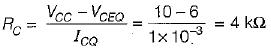Base current is,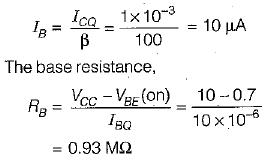QUESTION: 7

For the transistor circuit shown below,β = 100 and VBE(on) = 0.7 V.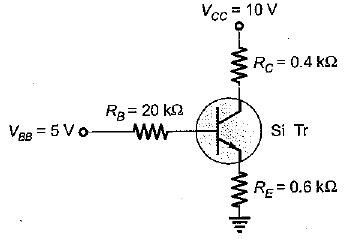The transistor is working under

Solution:

Let the transistor be operating under active region.
Applying KVL, we have: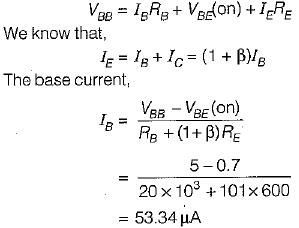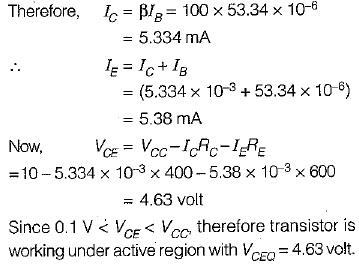QUESTION: 8

In a collector-to-base CE amplifier circuit having Vcc = 12 V, Rc = 250 Ω , IB = 0.25 mA, β = 100 and VCEQ = 8 V, the stability factor will be approximately given by (neglect VBE)

Solution:

For a coliector-to-base CE amplifier, stability factor is given by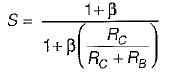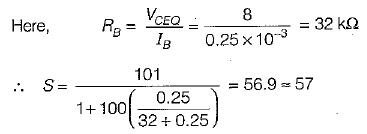QUESTION: 9

A self-bias circuit (with an emitter resiistor) stabilizes the__________  of the circuit against variations in temperature and process parameters.

Solution:

A self-bias circuit is used to establish a stable operating point in the self-biasing configuration. If Ic tends to increase, say, because Ico has risen as a result of an elevated temperature, the current in Re increases. As a consequence of the increase in voltage drop across Re, the base current is decreased. Hence, Ic will increase less than it would have been no self-biasing resistor Re.

QUESTION: 10

In typical circuits, the stabilization factor S = (ΔICICO) is

Solution:

For better stability of the circuit, stability factor S must be as small as possible.